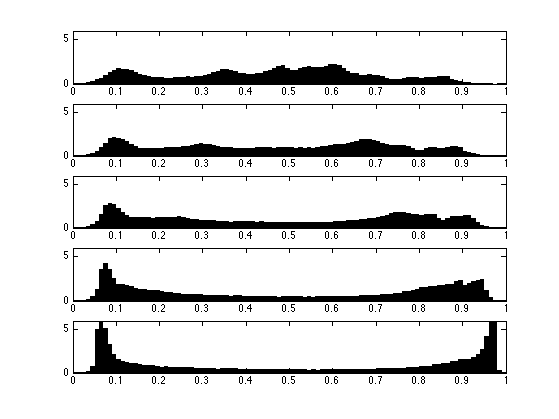$\newcommand{\NN}{\mathbb{N}} \newcommand{\CC}{\mathbb{C}} \newcommand{\GG}{\mathbb{G}} \newcommand{\LL}{\mathbb{L}} \newcommand{\PP}{\mathbb{P}} \newcommand{\QQ}{\mathbb{Q}} \newcommand{\RR}{\mathbb{R}} \newcommand{\VV}{\mathbb{V}} \newcommand{\ZZ}{\mathbb{Z}} \newcommand{\FF}{\mathbb{F}} \newcommand{\KK}{\mathbb{K}} \newcommand{\UU}{\mathbb{U}} \newcommand{\EE}{\mathbb{E}} \newcommand{\Aa}{\mathcal{A}} \newcommand{\Bb}{\mathcal{B}} \newcommand{\Cc}{\mathcal{C}} \newcommand{\Dd}{\mathcal{D}} \newcommand{\Ee}{\mathcal{E}} \newcommand{\Ff}{\mathcal{F}} \newcommand{\Gg}{\mathcal{G}} \newcommand{\Hh}{\mathcal{H}} \newcommand{\Ii}{\mathcal{I}} \newcommand{\Jj}{\mathcal{J}} \newcommand{\Kk}{\mathcal{K}} \newcommand{\Ll}{\mathcal{L}} \newcommand{\Mm}{\mathcal{M}} \newcommand{\Nn}{\mathcal{N}} \newcommand{\Oo}{\mathcal{O}} \newcommand{\Pp}{\mathcal{P}} \newcommand{\Qq}{\mathcal{Q}} \newcommand{\Rr}{\mathcal{R}} \newcommand{\Ss}{\mathcal{S}} \newcommand{\Tt}{\mathcal{T}} \newcommand{\Uu}{\mathcal{U}} \newcommand{\Vv}{\mathcal{V}} \newcommand{\Ww}{\mathcal{W}} \newcommand{\Xx}{\mathcal{X}} \newcommand{\Yy}{\mathcal{Y}} \newcommand{\Zz}{\mathcal{Z}} \newcommand{\al}{\alpha} \newcommand{\la}{\lambda} \newcommand{\ga}{\gamma} \newcommand{\Ga}{\Gamma} \newcommand{\La}{\Lambda} \newcommand{\Si}{\Sigma} \newcommand{\si}{\sigma} \newcommand{\be}{\beta} \newcommand{\de}{\delta} \newcommand{\De}{\Delta} \renewcommand{\phi}{\varphi} \renewcommand{\th}{\theta} \newcommand{\om}{\omega} \newcommand{\Om}{\Omega} \renewcommand{\epsilon}{\varepsilon} \newcommand{\Calpha}{\mathrm{C}^\al} \newcommand{\Cbeta}{\mathrm{C}^\be} \newcommand{\Cal}{\text{C}^\al} \newcommand{\Cdeux}{\text{C}^{2}} \newcommand{\Cun}{\text{C}^{1}} \newcommand{\Calt}{\text{C}^{#1}} \newcommand{\lun}{\ell^1} \newcommand{\ldeux}{\ell^2} \newcommand{\linf}{\ell^\infty} \newcommand{\ldeuxj}{{\ldeux_j}} \newcommand{\Lun}{\text{\upshape L}^1} \newcommand{\Ldeux}{\text{\upshape L}^2} \newcommand{\Lp}{\text{\upshape L}^p} \newcommand{\Lq}{\text{\upshape L}^q} \newcommand{\Linf}{\text{\upshape L}^\infty} \newcommand{\lzero}{\ell^0} \newcommand{\lp}{\ell^p} \renewcommand{\d}{\ins{d}} \newcommand{\Grad}{\text{Grad}} \newcommand{\grad}{\text{grad}} \renewcommand{\div}{\text{div}} \newcommand{\diag}{\text{diag}} \newcommand{\pd}{ \frac{ \partial #1}{\partial #2} } \newcommand{\pdd}{ \frac{ \partial^2 #1}{\partial #2^2} } \newcommand{\dotp}{\langle #1,\,#2\rangle} \newcommand{\norm}{|\!| #1 |\!|} \newcommand{\normi}{\norm{#1}_{\infty}} \newcommand{\normu}{\norm{#1}_{1}} \newcommand{\normz}{\norm{#1}_{0}} \newcommand{\abs}{\vert #1 \vert} \newcommand{\argmin}{\text{argmin}} \newcommand{\argmax}{\text{argmax}} \newcommand{\uargmin}{\underset{#1}{\argmin}\;} \newcommand{\uargmax}{\underset{#1}{\argmax}\;} \newcommand{\umin}{\underset{#1}{\min}\;} \newcommand{\umax}{\underset{#1}{\max}\;} \newcommand{\pa}{\left( #1 \right)} \newcommand{\choice}{ \left\{ \begin{array}{l} #1 \end{array} \right. } \newcommand{\enscond}{ \left\{ #1 \;:\; #2 \right\} } \newcommand{\qandq}{ \quad \text{and} \quad } \newcommand{\qqandqq}{ \qquad \text{and} \qquad } \newcommand{\qifq}{ \quad \text{if} \quad } \newcommand{\qqifqq}{ \qquad \text{if} \qquad } \newcommand{\qwhereq}{ \quad \text{where} \quad } \newcommand{\qqwhereqq}{ \qquad \text{where} \qquad } \newcommand{\qwithq}{ \quad \text{with} \quad } \newcommand{\qqwithqq}{ \qquad \text{with} \qquad } \newcommand{\qforq}{ \quad \text{for} \quad } \newcommand{\qqforqq}{ \qquad \text{for} \qquad } \newcommand{\qqsinceqq}{ \qquad \text{since} \qquad } \newcommand{\qsinceq}{ \quad \text{since} \quad } \newcommand{\qarrq}{\quad\Longrightarrow\quad} \newcommand{\qqarrqq}{\quad\Longrightarrow\quad} \newcommand{\qiffq}{\quad\Longleftrightarrow\quad} \newcommand{\qqiffqq}{\qquad\Longleftrightarrow\qquad} \newcommand{\qsubjq}{ \quad \text{subject to} \quad } \newcommand{\qqsubjqq}{ \qquad \text{subject to} \qquad }$

Optimal Transport in 1-D

# Optimal Transport in 1-D

This tour details the computation of discrete 1-D optimal transport with application to grayscale image histogram manipulations.

## Installing toolboxes and setting up the path.

You need to download the following files: signal toolbox and general toolbox.

You need to unzip these toolboxes in your working directory, so that you have toolbox_signal and toolbox_general in your directory.

For Scilab user: you must replace the Matlab comment '%' by its Scilab counterpart '//'.

Recommandation: You should create a text file named for instance numericaltour.sce (in Scilab) or numericaltour.m (in Matlab) to write all the Scilab/Matlab command you want to execute. Then, simply run exec('numericaltour.sce'); (in Scilab) or numericaltour; (in Matlab) to run the commands.

Execute this line only if you are using Matlab.

getd = @(p)path(p,path); % scilab users must *not* execute this


Then you can add the toolboxes to the path.

getd('toolbox_signal/');
getd('toolbox_general/');


## Optimal Transport and Assignement

We consider data $$f \in \RR^{N \times d}$$, that can corresponds for instance to an image of $$N$$ pixels, with $$d=1$$ for grayscale image and $$d=3$$ for color image. We denote $$f = (f_i)_{i=1}^N$$ with $$f_i \in \RR^d$$ the elements of the data.

The discrete (empirical) distribution in $$\RR^d$$ associated to this data $$f$$ is the sum of Diracs $\mu_f = \frac{1}{N} \sum_{i=1}^N \de_{f_i}.$

An optimal assignement between two such vectors $$f,g \in \RR^{N \times d}$$ is a permutation $$\si \in \Si_N$$ that minimizes $\si^\star \in \uargmin{\si \in \Si_N} \sum_{i=1}^N C(f_i,g_{\si(i)})$ where $$C(u,v) \in \RR$$ is some cost function.

In the following, we consider $$L^p$$ costs $\forall (u,v) \in \RR^d \times \RR^d, \quad C(u,v) = \norm{u-v}^p$ where $$\norm{\cdot}$$ is the Euclidean norm and $$p\geq 1$$.

This optimal assignement defines the $$L^p$$ Wasserstein distance between the associated point clouds distributions $W_p(\mu_f,\mu_g)^p = \sum_{i=1}^N \norm{f_i - g_{\si(i)}}^p = \norm{f - g \circ \si}_p^p$ where $$g \circ \si = (g_{\si(i)})_i$$ is the re-ordered points cloud.

## Grayscale Image Distribution

We consider here the case $$d=1$$, in which case one can compute easily the optimal assignement $$\si^\star$$.

Load an image $$f \in \RR^N$$ of $$N=n \times n$$ pixels.

n = 256;
f = rescale( load_image('lena', n) );


Display it.

clf;
imageplot(f);A convenient way to visualize the distribution $$\mu_f$$ is by computing an histogram $$h \in \RR^Q$$ composed using $$Q$$ bins $$[u_k,u_{k+1})$$. The histogram is computed as $\forall k=1,\ldots,Q, \quad h(p) = \abs{\enscond{i}{ f_i \in [u_k,u_{k+1}) }}.$

Number of bins.

Q = 50;


Compute the histogram.

[h,t] = hist(f(:), Q);


Display this normalized histogram. To make this curve an approximation of a continuous distribution, we normalize $$h$$ by $$Q/N$$.

clf;
bar(t,h*Q/n^2); axis('tight');Exercice 1: (check the solution) Compute and display the histogram of $$f$$ for an increasing number of bins.

exo1;Load another image $$g \in \RR^N$$.

g = rescale( mean(load_image('fingerprint', n),3) );


Display it.

clf;
imageplot(g);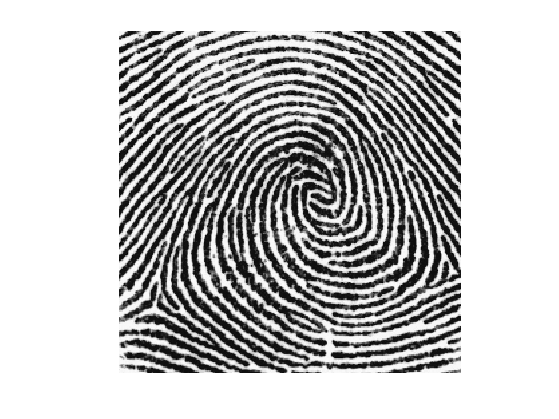Exercice 2: (check the solution) Compare the two histograms.

exo2;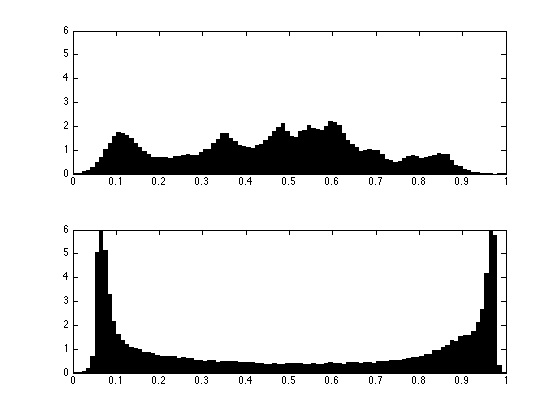## 1-D Optimal Assignement

For 1-D data, $$d=1$$, one can compute explicitely an optimal assignement $$\si^\star \in \Si_N$$ for any cost $$C(u,v) = \phi(\abs{u-v})$$ where $$\phi : \RR \rightarrow \RR$$ is a convex function. This is thus the case for the $$L^p$$ optimal transport.

This is obtained by computing two permutations $$\si_f, \si_g \in \Si_N$$ that order the values of the data $f_{\si_f(1)} \leq f_{\si_f(2)} \leq \ldots f_{\si_f(N)}$ $g_{\si_g(1)} \leq g_{\si_g(2)} \leq \ldots g_{\si_g(N)}.$

An optimal assignement is then optained by assigning, for each $$k$$, the index $$i = \si_f(k)$$ to the index $$\si_g(k)$$, i.e. $\si^\star = \si_g \circ \si_f^{-1}$ where $$\si_f^{-1}$$ is the inverse permutation, that satisfies $\si_f^{-1} \circ \si_f = \text{Id}$.

Note that this optimal assignement $$\si^\star$$ is not unique when there are two pixels in $$f$$ or $$g$$ having the same value.

Compute $$\si_f, \si_g$$ in $$O(N \log(N))$$ operations using a fast sorting algorithm (e.g. QuickSort).

[~,sigmaf] = sort(f(:));
[~,sigmag] = sort(g(:));


Compute the inverse permutation $$\sigma_f^{-1}$$.

sigmafi = [];
sigmafi(sigmaf) = 1:n^2;


Compute the optimal permutation $$\sigma^\star$$.

sigma = sigmag(sigmafi);


The optimal assignement is used to compute the projection on the set of image having the pixel distribution $$\mu_g$$ $\Hh_g = \enscond{m \in \RR^N}{ \mu_m = \mu_g }.$ Indeed, for any $$p > 1$$, the $$L^p$$ projector on this set $\pi_g( f ) = \uargmin{m \in \Hh_g} \norm{ f - m }_p$ is simply obtained by re-ordering the pixels of $$g$$ using an optimal assignement $$\si^\star \in \Si_N$$ between $$f$$ and $$g$$, i.e. $\pi_g( f ) = g \circ \si^\star.$

This projection $$\pi_g( f )$$ is called the histogram equalization of $$f$$ using the histogram of $$g$$

Compute the projection.

f1 = reshape(g(sigma), [n n]);


Check the new histogram.

clf;
[h,t] = hist(f1(:), p);
bar(t,h*p/n^2);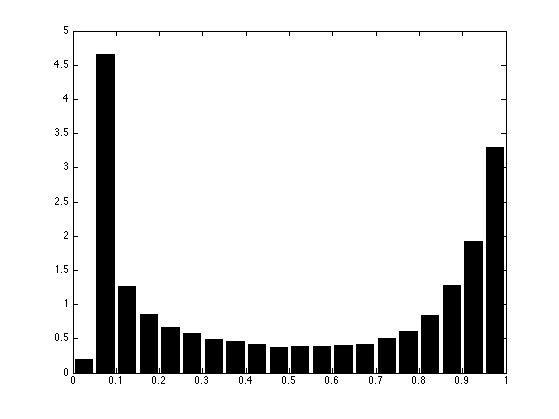Compare before/after equalization.

clf;
imageplot(f, 'f', 1,2,1);
imageplot(f1, '\pi_g(f)',  1,2,2);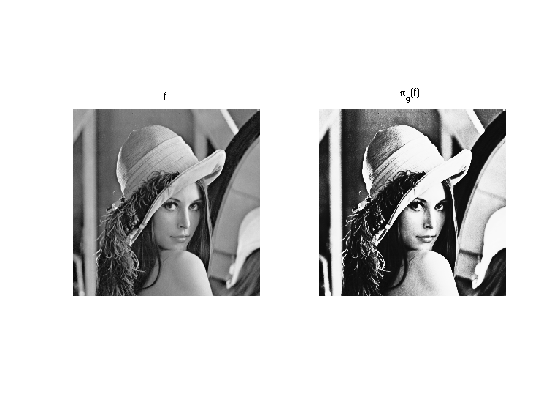## Histogram Interpolation

We now introduce the linearly interpolated image $\forall t \in [0,1], \quad f_t = (1-t) f + t g \circ \sigma^{\star} .$

One can show that the distribution $$\mu_{f_t}$$ is the geodesic interpolation in the $$L^2$$-Wasserstein space between the two distribution $$\mu_f$$ (obtained for $$t=0$$) and $$\mu_g$$ (obtained for $$t=1$$).

One can also show that it is the barycenter between the two distributions since it has the following variational characterization $\mu_{f_t} = \uargmin{\mu} (1-t)W_2(\mu_f,\mu)^2 + t W_2(\mu_g,\mu)^2 .$

Define the interpolation operator.

ft = @(t)reshape( t*f1 + (1-t)*f, [n n]);


The midway equalization is obtained for $$t=1/2$$.

clf;
imageplot(ft(1/2));Exercice 3: (check the solution) Display the progression of the interpolation of the histograms.

exo3;